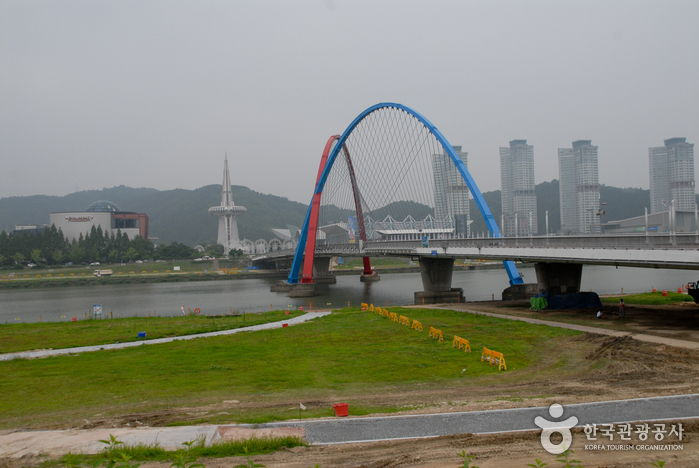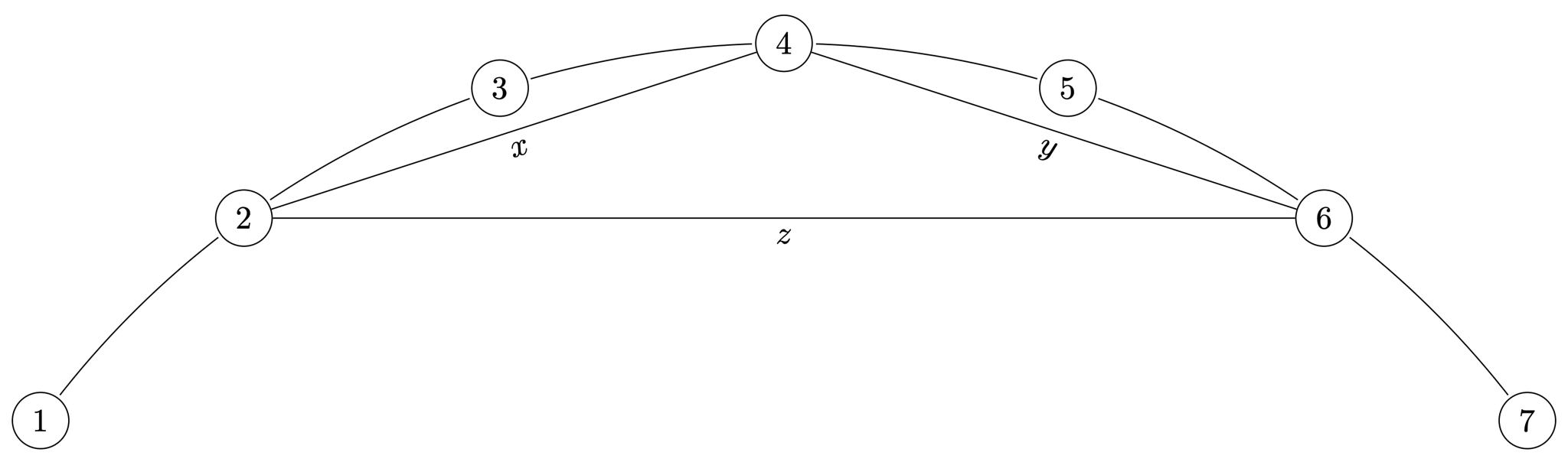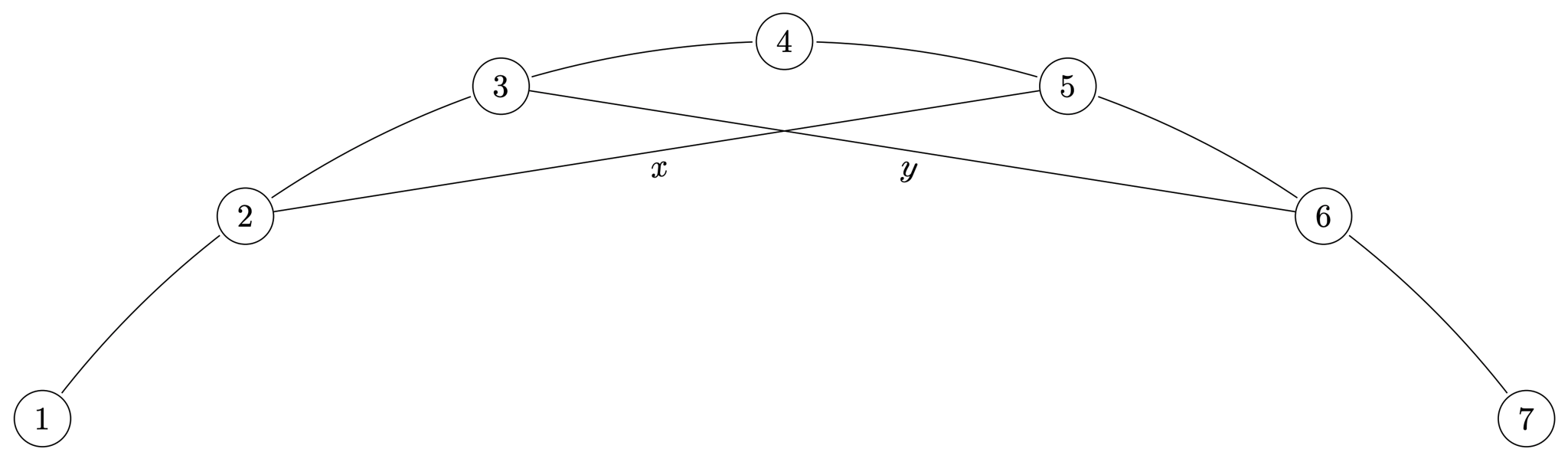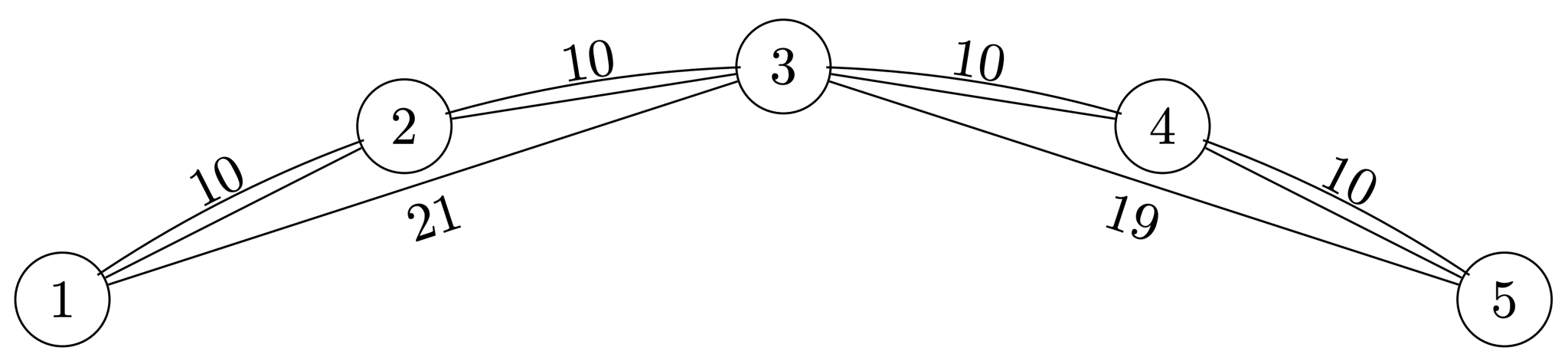시간 제한메모리 제한제출정답맞힌 사람정답 비율
1.5 초 (추가 시간 없음) 1024 MB138765651.376%

## 문제Figure 1. Gapcheon and an Expo bridge in a cloudy day

Gapcheon is a stream that flows through the Daedeok Innopolis: A research district in Daejeon which includes KAIST, Expo Science Park, National Science Museum, among many others. The waterfront of Gapcheon is used as a park, which is a facility for leisure and recreation.

In this problem, we model the Gapcheon as a slightly curved arc. In the arc, there are exactly $10^6$ points marked by each centimeter. In Gapcheon, there are $N$ bridges that connect two distinct points in the arc in a straight line segment. Such a line segment may touch other segments in an endpoint but never crosses them otherwise. For each pair of points, there exists at most one bridge that directly connects those two points.Figure 2. $x, y, z$ are bridges that do not cross but only touch each other in an endpoint. This can be a possible input instance. Points with number $8 \ldots 10^6$ are omitted for brevity.Figure 3. $x, y$ are bridges that cross each other. This is not a possible input instance. Points with number $8 \ldots 10^6$ are omitted for brevity.

The city council is planning to place some lights in the bridges, to make Gapcheon as a more enjoyable place in the night. For each bridge, the city council calculated the aesthetical value if the lights are installed in these bridges. These value can be represented as a positive integer.

However, too many lightings will annoy the residents at midnight. To address this issue, the council decided to make some regulations: for every arc between two adjacent points, there should be at most $k$ lighted bridges visible from there. We call a line segment visible from an arc connecting $i, i+1$, when one endpoint of the segment has an index at most $i$, and another endpoint of the segment has an index at least $i+1$.

The city council wants to consider the tradeoff between light pollution and the night view, so you should provide the maximum possible sum of aesthetical value, for all integers $1 \le k \le N$.

## 입력

The first line contains an integer $N$. ($1 \le N \le 250\,000$)

The next $N$ lines contain three integers $S_i, E_i, V_i$, which denotes there is a straight line bridge connecting points $S_i, E_i$, and having aesthetic value $V_i$. ($1 \le S_i < E_i \le 10^6, 1 \le V_i \le 10^9$).

It's guaranteed that no lines connect the same pair of points, and no two different line segments cross.

## 출력

Print $N$ integers separated by a space. The $i$-th integer ($1 \le i \le N$) should be the answer if $k = i$.

## 예제 입력 1

6
1 2 10
2 3 10
1 3 21
3 4 10
4 5 10
3 5 19


## 예제 출력 1

41 80 80 80 80 80


## 예제 입력 2

4
1 5 1
2 5 1
3 5 1
4 5 1


## 예제 출력 2

1 2 3 4


## 힌트Figure 4. Depiction of Sample Input 1.

Copyright notice for Figure 1:사진제공(한국관광공사 김지호)-한국관광공사